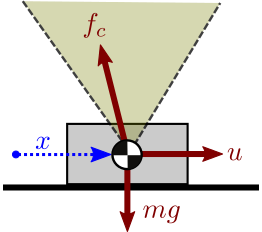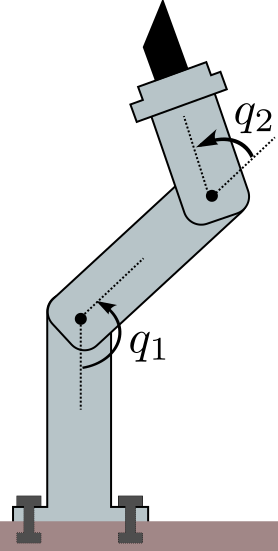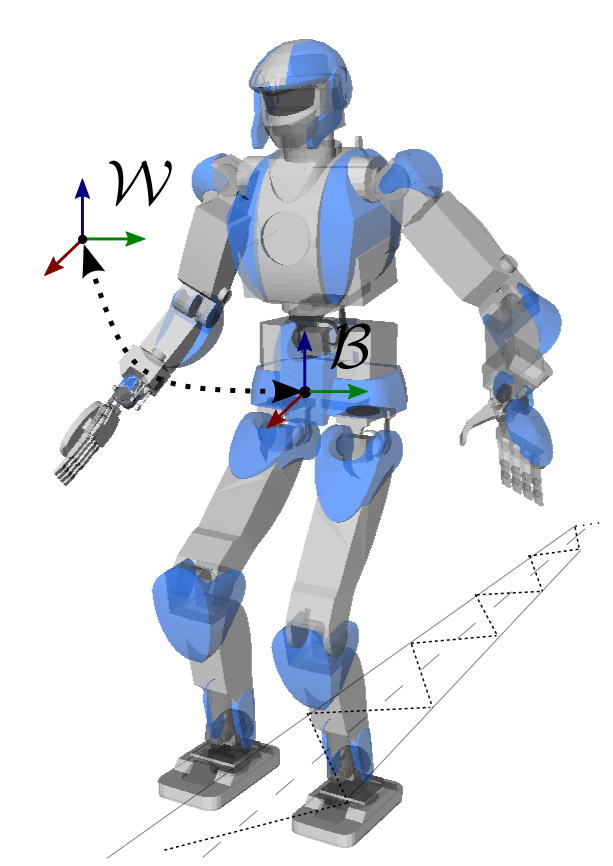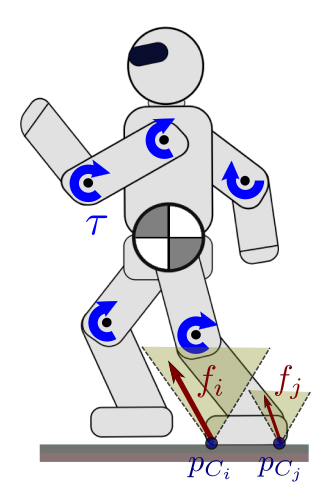# Equations of motion

It is common to see the equations of motion of manipulators or humanoids reminded in the preamble of research papers. Their origin can be tracked down in textbooks, where they are derived from Newtonian or Lagrangian mechanics. In this note, we will see what are the equations of motion for manipulators and legged robots, and how to compute them in practice.

## Definition

The equations of motion of a physical system describe its motion as a function of time and optional control inputs. In their general form, they are written:

\begin{equation*} F(\q(t), \qd(t), \qdd(t), \bfu(t), t) = 0, \end{equation*}

where

• $$t$$ is the time variable,
• $$\q$$ is the vector of generalized coordinates, for instance the vector of joint-angles for a manipulator,
• $$\qd$$ is the first time-derivative (velocity) of $$\q,$$
• $$\qdd$$ is the second time-derivative (acceleration) of $$\q,$$
• $$\bfu$$ is the vector of control inputs.

These equations provide a mapping between the control space, the commands that we send to actuators, and the state space of robot motions.

### Example

Imagine a rigid block sliding on a table, which we can see as a 1-DOF (one degree of freedom) system with coordinate $$x$$. The operator applies a horizontal force $$u$$ that pushes the block forward.If the force is high enough to overcome friction, applying Newton's second law of motion (and Coulomb's model of sliding friction) we get: $$m \xdd = u - \mu_k m g$$ with $$\mu_k$$ the kinetic coefficient of friction. This expression is of the form $$F(x, \xd, \xdd, u, t) = 0.$$

### Assumptions

In robotics, we usually make the following assumptions:

• Rigid bodies: the robot consists of a set of rigid bodies, called "links", connected by actuated joints. This assumption allows us to use the theory of rigid body dynamics, which is central to both manipulator and humanoid robotics.
• D'Alembert's principle: internal forces are conservative. Internal forces, also known as constraint forces, are the forces acting at each joint to prevent motions that are not alongside the joint axis. Assuming that they are conservative is equivalent to Gauss's principle of least constraint.

## Case of manipulatorsManipulators are a particular type of articulated system where at least one link is fixed to the environment. We suppose that the constraint is strong enough to withhold any effort exerted on it. Under these assumptions, the equations of motion for a manipulator can be written:

\begin{equation*} \bfM(\bfq) \qdd + \qd^\top \bfC(\bfq) \qd = \bftau + \bftau_g(\bfq) \end{equation*}

where, denoting by $$n = \dim(\bfq)$$ the degree of freedom of the robot,

• $$\bfM(\q)$$ is the $$n \times n$$ inertia matrix,
• $$\bfC(\q)$$ is the $$n \times n \times n$$ Coriolis tensor,
• $$\bftau$$ is the $$n$$-dimensional vector of actuated joint torques,
• $$\bftau_g(\q)$$ is the $$n$$-dimensional vector of external joint torques caused by gravity.

Contrary to the general form $$F(\q, \qd, \qdd, \bftau, t) = 0$$, this expression is time-invariant: time $$t$$ does not appear in the formula, so that the current state $$(\q, \qd)$$ and applied joint torque $$\bftau$$ we can directly compute the resulting joint accelerations $$\qdd$$ (this operation is known as forward dynamics). The system has no memory: regardless of how it got there, given its current state and joint torques, it will always accelerate in the same way.

### Inertia matrix and Coriolis tensor

The inertia matrix and Coriolis tensor can be derived from link inertias and their Jacobians by:

\begin{align} \bfM(\bfq) & = \sum_i \bfJ^t_i(\bfq)^\top m_i \bfJ^t_i(\bfq) + \bfJ^R_i(\bfq)^\top \bfI_i \bfJ^R_i(\bfq) \\ \qd^\top \bfC(\bfq) & = \sum_i \bfJ^t_i(\bfq)^\top m_i \dot{\bfJ}^t_i(\bfq) + \bfJ^R_i(\bfq)^\top \bfI_i \dot{\bfJ}^R_i(\bfq) - \bfJ^R_i(\bfq)^\top (\bfI_i \bfJ^R_i \qd) \times \bfJ^R_i \end{align}

where $$m_i$$ is the total mass of link $$i$$, $$\bfI_i$$ is its inertia matrix, and the Jacobian $$\bfJ_i$$ of the frame attached to link $$i$$ stacks:

• the rotational Jacobian $$\bfJ^{R}_i$$ such that the angular velocity $$\omega_i$$ of the link frame with respect to the inertial frame satisfies $$\omega_i = \bfJ^{R}_i \qd$$
• the translational Jacobian $$\bfJ^{t}_{\calC}$$ such that the linear velocity $$\bfpd_i$$ of the origin of the link frame (in the inertial frame) satisfies $$\bfpd_i = \bfJ^{t}_i \qd$$.

In OpenRAVE, the former is for instance computed by Robot.ComputeJacobianAxisAngle and the latter by Robot.ComputeJacobianTranslation. These formulas are good to keep in mind, but there are more efficient techniques to compute the inertia matrix in practice. See Wieber's comments on the structure of the dynamics of articulated motion for their derivation from Gauss's principle of least constraint.

### Gravity torques and external forces

External forces applied to the system can be expressed via a similar formula:

\begin{equation*} \bftau_{\mathit{ext}}(\bfq) = \sum_i \bfJ^t_i(\bfq)^\top \bff_{\mathit{ext},i} + \bfJ^R_i(\bfq)^\top \bftau_{\mathit{ext},i} \end{equation*}

where $$\bff_{\mathit{ext},i}$$ denotes the resultant of external forces applied to link $$i$$ of the robot, and $$\bftau_{\mathit{ext},i}$$ is the moment of these forces. Together, they form the external wrench applied to link $$i$$. Wrench coordinates should be consistent with the definitions of our Jacobian matrices: we assumed above that the image of our Jacobian matrices are expressed in the inertial frame, therefore wrench coordinates should be expressed in the inertial frame as well.

Let us consider gravity as our external force. Assuming that the frame attached to link $$i$$ is located at the link's center of mass, joint torques caused by gravity can be written as:

\begin{equation*} \bftau_{g}(\bfq) = \sum_i m_i \bfJ^t_i(\bfq)^\top \bfg \end{equation*}

By definition, the Jacobian $$\bfJ_\mathit{com}$$ of the center of mass is such that $$\sum_i m_i \bfJ_\mathit{com} = \sum_i m_i \bfJ^t_i$$. We can therefore condense the formula above as $$\bftau_g(\bfq) = m \bfJ_\mathit{com}(\bfq) \bfg$$.

## Case of legged robots

Contrary to manipulators, legged robots such as humanoids can make or break contacts with the environment. To fully describe their position in space, the vector of generalized coordinates consists of not only the vector of joint angles, but also six more coordinates that describe the position and orientation of the floating base frame $${\cal B}$$ (also known as base link frame) with respect to the inertial frame $${\cal W}$$. If the robot has $$n$$ actuated joints, this means that $$\q \in \mathbb{R}^{n + 6}$$. As the vector of actuated torques is still $$\bftau \in \mathbb{R}^n$$, humanoids are underactuated systems: they cannot control directly all their degrees of freedom.With manipulators, the contact constraint with the environment was implicitely enforced, as the vector of joint angles was independent from the world position of the robot. With legged robots it is not the case any more, and contacts need to be actively enforced by kinematic contact constraints.

### Point contacts and contact forcesKinematically, a point contact constraint is an equality of the form

\begin{equation*} \bfp_{C}(\q) = \bfp_{D}, \end{equation*}

where $$C$$ is the position of a robot's point in the inertial frame and $$D$$ is a fixed point (always in the inertial frame unless written otherwise). It binds positions, but also velocities and accelerations by taking time derivatives:

\begin{equation*} \begin{array}{rcl} \bfpd_C = 0 & \Leftrightarrow & \bfJ_C(\bfq) \qd = \boldsymbol{0} \\ \bfpdd_C = 0 & \Leftrightarrow & \bfJ_C(\bfq) \qdd + \qd^\top \bfH_C(\bfq) \qd = \boldsymbol{0} \end{array} \end{equation*}

Here $$\bfJ_C = ({\partial \bfp_C} / {\partial \bfq})$$ is the Jacobian matrix of the contact constraint, while $$\bfH_C = ({\partial^2 \bfp_C} / {\partial \bfq^2})$$ is its Hessian.

Assuming the legged robot is making $$k$$ such contacts $$C_1, \ldots, C_k$$ with its environment, its equations of motion become:

\begin{equation*} \bfM(\bfq) \qdd + \qd^\top \bfC(\bfq) \qd = \bfS^\top \bftau + \bftau_g(\bfq) + \sum_{i=1}^k \bfJ_{C_i}^\top \bff_i, \end{equation*}

where:

• $$\bfS$$ is the $$n \times (n + 6)$$ matrix that selects the $$n$$ actuated coordinates in $$\q$$. If the six floating base coordinates are the last entries of $$\q,$$ then $$\bfS = \left[ \bfE_n \ {\boldsymbol{0}}_{6 \times n} \right]$$.
• $$\bfJ_{C_i}$$ is jacobian of the position vector (in the inertial frame) $$\bfp_{C_i}(\q)$$ of a contact point $$C_i.$$
• $$\bff_i$$ is the contact force exerted by the environment on the robot at the contact point $$C_i.$$

The sum over contacts is usually compressed as $$\bfJ_C^\top \bff$$ by stacking force vectors $$\bff_i$$ (resp. Jacobian matrices $$\bfJ_{C_i}$$) vertically into $$\bff$$ (resp. $$\bfJ_C$$). See the follow-up page on the constrained equations of motion for details on how these Jacobian-transpose terms derive from Gauss's principle of least constraint.

Contact points $$C_i$$ are taken at the interface between robot's links and the environment. In the case of surface contacts, when a robot's surface is in full contact with an environment surface (such as a foot on the floor), it is sufficient to take $$C_i$$'s at the vertices of the contact polygon. This is shown for instance in Section III of this paper.

### Surface contacts and contact wrenches

Surface contacts, such as a flat foot on the floor, can be modeled by using three or more point contacts, but this redundant representation is redundant. A non-redundant alternative to this is to use a more general frame contact constraints.

Kinematically, a surface contact constraint is an equality

\begin{equation*} {}^{\cal W}\bfT_{\cal C}(\bfq) = \bfE_4, \end{equation*}

where $$\bfE_4$$ is the $$4 \times 4$$ identity matrix and $${}^{\calW}\bfT_{\calC}$$ is the transform matrix between a robot's contact frame $$\cal C$$ and the inertial frame $$\cal W$$. (If you are not familiar with kinematic transforms, check out for instance Chapter 2 of Kajita et al. (2014).) The origin of the contact frame can be a contact point $$C$$, and its orientation is parallel to that of the contacting link.

The kinematic contact constraints binds velocities and accelerations as well by:

\begin{equation*} \begin{array}{rcl} \frac{\rm d}{\d{t}} {}^{\calW}\bfT_\calC = \boldsymbol{0}_{4 \times 4} & \Leftrightarrow & \bfJ_{\cal C} \qd = \boldsymbol{0} \\ \frac{\rm d^2}{\d{t}^2} {}^{\calW}\bfT_\calC = \boldsymbol{0}_{4 \times 4} & \Leftrightarrow & \bfJ_{\cal C} \qdd + \qd^\top \bfH_{\calC} \qd = \boldsymbol{0} \end{array} \end{equation*}

where $$\bfJ_{\cal C}$$ is the Jacobian of the contact frame $$\cal C$$ obtained by stacking its rotational and translational Jacobians.

Under surface contact constraints, the equations of motion become:

\begin{equation*} \bfM(\bfq) \qdd + \qd^\top \bfC(\bfq) \qd = \bfS^\top \bftau + \bftau_g(\bfq) + \sum_{i=1}^N \bfJ_{\calC_i}^{\top} \bfw_i, \end{equation*}

where $$\bfw_i$$ is the contact wrench exerted by the environment on the robot at the contacting link $$i$$. See Chapter 2 of Roy Featherstone's Rigid Body Dynamics Algorithms for an introduction to wrenches and, more generally, to spatial vector algebra. In vector form, $$\bfw_i$$ stacks the resultant $$\bff_i$$ of contact forces and the moment $$\bfm_i$$ of these forces around the origin $$C_i$$ of the contact frame. The order of $$\bfm_i$$ and $$\bff_i$$ does not matter, as long as it is consistent with the contact frame Jacobian.

## To go further

My favorite introduction to equations of motion is Wieber's Some comments on the structure of the dynamics of articulated motion, which I warmly recommend. It is one of the rare papers where the formulas for $$\bfM(q)$$ and $$\bfC(\bfq)$$ are written down in plain and understandable form from link inertias and Jacobian matrices. See the follow-up page on the constrained equations of motion for more details on this.

There is a particular choice of generalized to simplifies the formula of the gravity torque vector. It consists in taking the coordinates of the center of mass of the system as as translation coordinate for the floating base. With such generalized coordinates $$\tilde{\bfq}$$, the equations of motion become:

\begin{equation*} \bfM(\tilde{\bfq}) \ddot{\tilde{\bfq}} + \dot{\tilde{\bfq}}^\top \bfC(\tilde{\bfq}) \dot{\tilde{\bfq}} = \begin{bmatrix} m \bfg \\ \bfzero_{n + 3} \end{bmatrix} + \bfS^\top \bftau + \bfJ^\top \bff + \bftau_\mathrm{ext} \end{equation*}

Check out Garofalo et al. (2015) for a derivation of this property.

When using contact points and their corresponding contact forces, the contact stability condition to avoid slippage is that contact forces stay inside their respective Coulomb friction cones. This condition generalizes to contact frames and their corresponding contact wrenches: in this case, Coulomb friction generalizes to wrench friction cones. If wrenches are new to you, you might want to learn more about screw theory which is commonly applied in robotics.

## Discussion

You can use Markdown with $\LaTeX$ formulas in your comment.